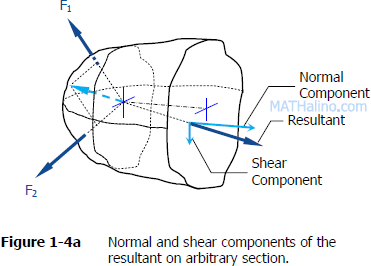## Solution to Problem 212 Axial Deformation

Problem 212
The rigid bar ABC shown in Fig. P-212 is hinged at A and supported by a steel rod at B. Determine the largest load P that can be applied at C if the stress in the steel rod is limited to 30 ksi and the vertical movement of end C must not exceed 0.10 in.## Solution to Problem 130 Bearing Stress

Problem 130
Figure P-130 shows a roof truss and the detail of the riveted connection at joint B. Using allowable stresses of τ = 70 MPa and σb= 140 MPa, how many 19-mm-diameter rivets are required to fasten member BC to the gusset plate? Member BE? What is the largest average tensile or compressive stress in BC and BE?## Solution to Problem 123 Shear Stress

Problem 123
A rectangular piece of wood, 50 mm by 100 mm in cross section, is used as a compression block shown in Fig. P-123. Determine the axial force P that can be safely applied to the block if the compressive stress in wood is limited to 20 MN/m2 and the shearing stress parallel to the grain is limited to 5MN/m2. The grain makes an angle of 20° with the horizontal, as shown. (Hint: Use the results in Problem 122.)Solution to Problem 122 Shear Stress Jhun Vert Mon, 04/20/2020 - 01:33 pm

Problem 122
Two blocks of wood, width w and thickness t, are glued together along the joint inclined at the angle θ as shown in Fig. P-122. Using the free-body diagram concept in Fig. 1-4a, show that the shearing stress on the glued joint is τ = P sin 2θ / 2A, where A is the cross-sectional area.## Solution to Problem 114 Normal Stress

Problem 114
The homogeneous bar ABCD shown in Fig. P-114 is supported by a cable that runs from A to B around the smooth peg at E, a vertical cable at C, and a smooth inclined surface at D. Determine the mass of the heaviest bar that can be supported if the stress in each cable is limited to 100 MPa. The area of the cable AB is 250 mm2 and that of the cable at C is 300 mm2.## Solution to Problem 113 Normal Stress

Problem 113
Find the stresses in members BC, BD, and CF for the truss shown in Fig. P-113. Indicate the tension or compression. The cross sectional area of each member is 1600 mm2.## Solution to Problem 112 Normal Stress

Problem 112
Determine the cross-sectional areas of members AG, BC, and CE for the truss shown in Fig. P-112. The stresses are not to exceed 20 ksi in tension and 14 ksi in compression. A reduced stress in compression is specified to reduce the danger of buckling.## Solution to Problem 111 Normal Stress

Problem 111
For the truss shown in Fig. P-111, calculate the stresses in members CE, DE, and DF. The cross-sectional area of each member is 1.8 in2. Indicate tension (T) or compression (C).